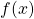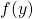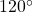# On the Diophantine equations z2 = f(x)2 ± f(x)f(y) + f(y)2

Qiongzhi Tang
Notes on Number Theory and Discrete Mathematics
Print ISSN 1310–5132, Online ISSN 2367–8275
Volume 27, 2021, Number 2, Pages 88—100
DOI: 10.7546/nntdm.2021.27.2.88-100

## Details

### Authors and affiliations

Qiongzhi TangSchool of Mathematics and Statistics, Changsha University of Science and Technology,
Hunan Provincial Key Laboratory of Mathematical Modeling and Analysis in Engineering
Changsha, 410114, China

### Abstract

Using the theory of Pell equation, we study the non-trivial positive integer solutions of the Diophantine equationsfor certain polynomials, which mean to construct integral triangles with two sides given by the values of polynomialsandwith the intersection angleor.

### Keywords

• Diophantine equation
• Pell equation
• Positive integer solution

• 11D25
• 11D72

### References

1. Burn, B. (2003). Triangles with a 60◦ angle and sides of integer length. The Mathematical Gazette, 87(508), 148–153.
2. Dickson, L. E. (2005). History of the Theory of Numbers, Vol. II: Diophantine Analysis. Dover Publications.
3. Gilder, J. (1982). Integer-sided triangles with an angle of 60◦. The Mathematical Gazette, 66(438), 261–266.
4. He, B., Togbé, A., & Ulas, M. (2010). On the Diophantine equation z2 = f(x)2 ± f(y)2, II. Bulletin of the Australian Mathematical Society, 82(2), 187–204.
5. Pocklington, H. C. (1914). Some Diophantine impossibilities. Mathematical Proceedings of the Cambridge Philosophical Society, 17, 110–118.
6. Read, E. (2006). On integer-sided triangles containing angles of 120◦ or 60◦. The Mathematical Gazette, 90(518), 299–305.
7. Selkirk, K. (1983). Integer-sided triangles with an angle of 120◦. The Mathematical Gazette, 67(442), 251–255.
8. Sierpinski, W. (1962). Triangular Numbers. Biblioteczka Matematyczna 12, Warszawa (in Polish).
9. Tengely, Sz., & Ulas, M. (2017). On certain Diophantine equations of the form z2 = f(x)2±g(y)2. Journal of Number Theory, 174, 239–257.
10. Ulas, M., & Togbé, A. (2010). On the Diophantine equation z2 = f(x)2 ± f(y)2.
Publicationes Mathematicae Debrecen, 76(1–2), 183–201.
11. Youmbai, A. E. A., & Behloul, D. (2019). Rational solutions of the Diophantine equations f(x)2 ± f(y)2 = z2. Periodica Mathematica Hungarica, 79(2), 255–260.
12. Zhang, Y., & Tang, Q. Z. (2021). On the integer solutions of the Diophantine equations z2 = f(x)2 ± f(y)2. Periodica Mathematica Hungarica, accepted.
13. Zhang, Y., Tang, Q. Z., & Zhang, Y. N. (2020). On the Diophantine equations z2 = f(x)2 ± f(y)2 involving Laurent polynomials, II. submitted.
14. Zhang, Y., & Zargar, A. S. (2019). On the Diophantine equations z2 = f(x)2 ± f(y)2
involving quartic polynomials. Periodica Mathematica Hungarica, 79(1), 25–31.
15. Zhang, Y., & Zargar, A. S. (2020). On the Diophantine equations z2 = f(x)2 ± f(y)2 involving Laurent polynomials. Functiones et Approximatio, Commentarii Mathematici, 62(2), 187–201.

## Cite this paper

Tang, Q. (2021). On the Diophantine equations z2 = f(x)2 ± f(x)f(y) + f(y)2. Notes on Number Theory and Discrete Mathematics, 27(2), 88-100, doi: 10.7546/nntdm.2021.27.2.88-100.